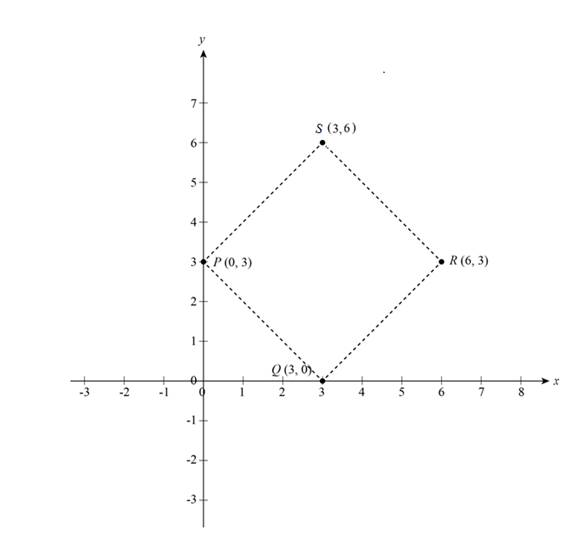# The point S of a square PQRS and plot points in the coordinate plane.### Precalculus: Mathematics for Calcu...

6th Edition
Stewart + 5 others
Publisher: Cengage Learning
ISBN: 9780840068071### Precalculus: Mathematics for Calcu...

6th Edition
Stewart + 5 others
Publisher: Cengage Learning
ISBN: 9780840068071

#### Solutions

Chapter 1, Problem 15T

(a)

To determine

## To evaluate: The point S of a square PQRS and plot points in the coordinate plane.

Expert Solution

The point S is (3,6).

### Explanation of Solution

Given:

The coordinates of points of square PQRS is P(0,3), Q(3,0) and R(6,3).

Formula used:

The distance formula of two points A(x1,y1) and B(x2,y2) is,

d(A,B)=(x2x1)2+(y2y1)2 (1)

Where, d is the distance between two points and x1, x2, y1 and y2 are coordinates of two points.

Calculation:

Let the coordinates of points be S(x,y).

Find the distance between the points P(0,3) and Q(3,0).

Substitute 3 for x2, 0 for x1, 0 for y2 and 3 for y1 in formula (1).

d(P,Q)=(30)2+(03)2=9+9=18=32

The distance between two points R(6,3) and S(x,y) give another side of square.

Substitute x for x2, 6 for x1, y for y2 and 3 for y1 in formula (1).

d(R,S)=(x6)2+(y3)2=x212x+36+y26y+9=x2+y212x6y+45

Since all sides of square are equal in length, d(P,Q)=d(R,S).

Substitute 32 for d(R,S) in d(P,Q)=d(R,S).

(x2+y212x6y+45)2=(322)x2+y212x6y+45=18x2+y212x6y+27=0

From the above expression the equation is,

x2+y212x6y+27=0 (1)

Substitute 18 for d(P,S) to get the distance between point P(0,3) and S(x,y).

(x0)2+(y3)2=d(P,S)x2+y26y+9=(18)2x2+y26y9=0

The equation from above expression is,

x2+y26y9=0 (2)

Subtract equation (2) from equation (1) to get the values of x,

x2+y212x6y+27=0x2+y26y9=0_12x+36=0x=3

Substitute 3 for x in equation (2) to get value of y.

(3)2+y26y9=0y26y=0y(y6)=0y=0,6

These values of x- and y-coordinates gives the point S.

Since the point (3,0) exists already in square, so take y=6 in point S(x,y).

Thus, the coordinates of point S is (3,6)

Now the point S(3,6) is obtained.

In point P(0,3), x-coordinate is zero, so it is lies in y-axis similarly point Q(3,0) lies on x-axis and points R(6,3), S(3,6) both coordinates are positive so it is lies in I Quadrant.

The coordinate plane of points of square is shown below in Figure (1).Figure (1)

From Figure (1), it is observed that the coordinates of the square PQRS are P(0,3), Q(3,0) and R(6,3) and S(3,6).

(b)

To determine

### To find: The area of square PQRS.

Expert Solution

The area of square PQRS is 18.

### Explanation of Solution

Given:

The coordinates of points of square PQRS is P(0,3), Q(3,0), R(6,3).

Calculation:

The formula of area of square is,

area=(side)2

From part (a) the side of square PQRS is 32.

Substitute 32 for side in the formula of area of square.

Area=(32)2=9(2)=18

Thus, the area of square PQRS is 18.

### Have a homework question?

Subscribe to bartleby learn! Ask subject matter experts 30 homework questions each month. Plus, you’ll have access to millions of step-by-step textbook answers!